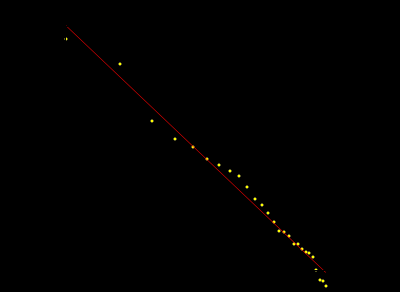M A T H 2 1 B
Mathematics Math21b Spring 2009
Linear Algebra and Differential Equations
Exhibit: Linear algebra: zipf lawHere is a nice data fitting problem which empirically leads to Zipfs law. If we read in the data of the population of cities: ```A=ReadList["data.txt","Number"]; B=Partition[A,2]; n=Length[B]; B1=Table[{Log[k],Log[B[[k,1]]]},{k,27}]; Fit[B1,{1,x},x] ``` and do a linear fit, we measure a power law. The linear fit is 17-0.77 k as mentioned in the New York Times article New York Times: Math and the city. Note that the law only applies to the top. If we take all data, we get 17.5-0.95 k: ```A=ReadList["data.txt","Number"]; B=Partition[A,2]; n=Length[B]; B1=Table[{Log[k],Log[B[[k,1]]]},{k,100}]; Fit[B1,{1,x},x] ``` and a quadratic fit is probably better ```A=ReadList["data.txt","Number"]; B=Partition[A,2]; n=Length[B]; B1=Table[{Log[k],Log[B[[k,1]]]},{k,n}]; Fit[B1,{1,x,x^2},x] ```